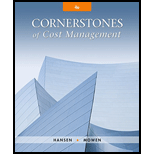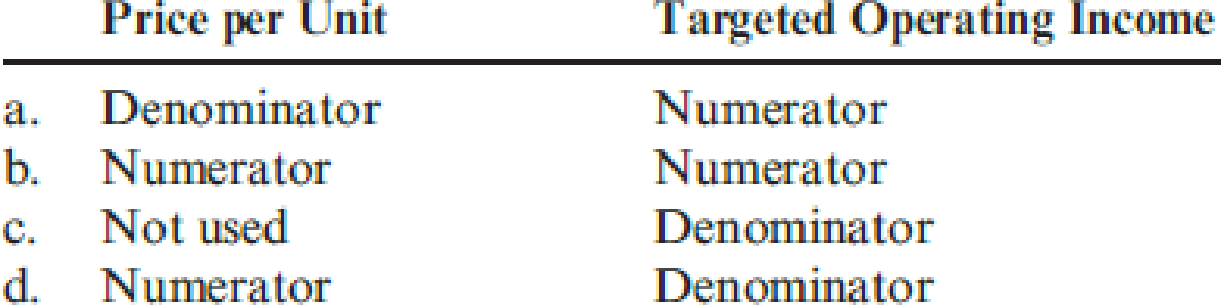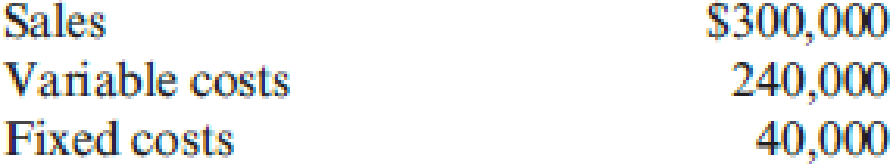# Choose the best answer for each of the following multiple-choice questions. 1. Cost-volume-profit analysis includes some simplifying assumptions. Which of the following is not one of these assumptions? a. Cost and revenues are predictable. b. Cost and revenues are linear over the relevant range. c. Changes in beginning and ending inventory levels are insignificant in amount. d. Sales mix changes are irrelevant. 2. The term relevant range , as used in cost accounting, means the range a. over which costs may fluctuate b. over which cost relationships are valid c. of probable production d. over which production has occurred in the past 10 years 3. How would the following be used in calculating the number of units that must be sold to earn a targeted operating income? 4. Information concerning Korian Corporation’s product is as follows: Assuming that Korian increased sales of the product by 20 percent, what should the operating income be? a. $20,000 b.$24,000 c. $32,000 d.$80,000 5. The following data apply to McNally Company for last year: McNally wants to sell an additional 50,000 units at the same selling price and contribution margin. By how much can fixed costs increase to generate additional profit equal to 10 percent of the sales value of the additional 50,000 units to be sold? a. $50,000 b.$57,500 c. $67,500 d.$125,000 6. Bryan Company’s break-even point is 8,500 units. Variable cost per unit is $140, and total fixed costs are$297,500 per year. What price does Bryan charge? a. $140 b.$35 c. $175 d. Cannot be determined from the above dataBuyFindarrow_forward ### Cornerstones of Cost Management (C... 4th Edition Don R. Hansen + 1 other Publisher: Cengage Learning ISBN: 9781305970663 #### Solutions Chapter SectionBuyFindarrow_forward ### Cornerstones of Cost Management (C... 4th Edition Don R. Hansen + 1 other Publisher: Cengage Learning ISBN: 9781305970663 Chapter 16, Problem 19E Textbook Problem 169 views ## Choose the best answer for each of the following multiple-choice questions. 1. Cost-volume-profit analysis includes some simplifying assumptions. Which of the following is not one of these assumptions? a. Cost and revenues are predictable. b. Cost and revenues are linear over the relevant range. c. Changes in beginning and ending inventory levels are insignificant in amount. d. Sales mix changes are irrelevant. 2. The term relevant range, as used in cost accounting, means the range a. over which costs may fluctuate b. over which cost relationships are valid c. of probable production d. over which production has occurred in the past 10 years 3. How would the following be used in calculating the number of units that must be sold to earn a targeted operating income?4. Information concerning Korian Corporation’s product is as follows:Assuming that Korian increased sales of the product by 20 percent, what should the operating income be? a.$20,000 b. $24,000 c.$32,000 d. $80,000 5. The following data apply to McNally Company for last year:McNally wants to sell an additional 50,000 units at the same selling price and contribution margin. By how much can fixed costs increase to generate additional profit equal to 10 percent of the sales value of the additional 50,000 units to be sold? a.$50,000 b. $57,500 c.$67,500 d. $125,000 6. Bryan Company’s break-even point is 8,500 units. Variable cost per unit is$140, and total fixed costs are $297,500 per year. What price does Bryan charge? a.$140 b. $35 c.$175 d. Cannot be determined from the above data

1.

To determine

Identify the statement which is not an assumption of CVP analysis.

### Explanation of Solution

Cost Volume Profit Analysis (CVP Analysis): The Cost volume profit (CVP) analysis is helpful in determining how any type of change in cost determines company’s income.

Sales mix: Sales mix refers to relative distribution of the total sales amongst the total number of units sold by a company. It is also expressed as a percentage of units sold for each product produced with respect to the total units sold for all the products produced.

Option (d):

Sales mix plays a vital role in CVP analysis...

2.

To determine

Identify the meaning of relevant range.

3.

To determine

Identify the formula to compute the number of units to be sold to generate the target operating income.

4.

To determine

Identify the operating income if the sales is increased by 20%.

5.

To determine

Identify the new fixed cost.

6.

To determine

Identify the price per unit.

### Still sussing out bartleby?

Check out a sample textbook solution.

See a sample solution

#### The Solution to Your Study Problems

Bartleby provides explanations to thousands of textbook problems written by our experts, many with advanced degrees!

Get Started

Find more solutions based on key concepts
In what ways is economics a science?

Principles of Microeconomics (MindTap Course List)

Why is the American economy called a mixed economy?

Foundations of Business (MindTap Course List)

Would you agree that computerized corporate planning models were a fad during the 1990s but that because of a n...

Fundamentals of Financial Management, Concise Edition (with Thomson ONE - Business School Edition, 1 term (6 months) Printed Access Card) (MindTap Course List)